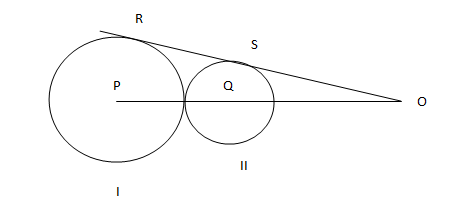### CAT 2004 Question Paper Question 3

Instructions

Directions for the following three questions: Answer the questions on the basis of the information given belowIn the adjoining figure I and II are circles with centres P and Q respectively, The two circles touch each other and have common tangent that touches them at points R and S respectively. This common tangent meets the line joining P and Q at O. The diameters of I and II are in the ratio 4: 3. It is also known that the length of PO is 28 cm.

Question 3

# The length of SO is[CAT 2004]

Solution

Triangles PRO and QSO are similar.

PR/QS = 4/3.

Therefore, PO/QO = 4/3 =>

Let the radius of 2 circles be 4R and 3R respectively.

28/(28-7R) = 4/3

4-R = 3

R = 1

Therefore, SO = $$\sqrt{21^2 - 3^2}$$ = $$\sqrt{432}$$ = $$12 \sqrt{3}$$ cm